This section is Section 2 of 3.

Speed as well as accuracy is important in this section. Work quickly, or you might not finish the paper. There are no  penalties for incorrect responses, only marks for correct answers, so you should attempt all 27 questions. Each question is worth one mark.

You must complete the answers within the time limit. Calculators are NOT permitted.

Good Luck!

Note – if press “End Exam” you can access full worked solutions for all past paper questions

The diagram shows some stages involved in genetic engineering.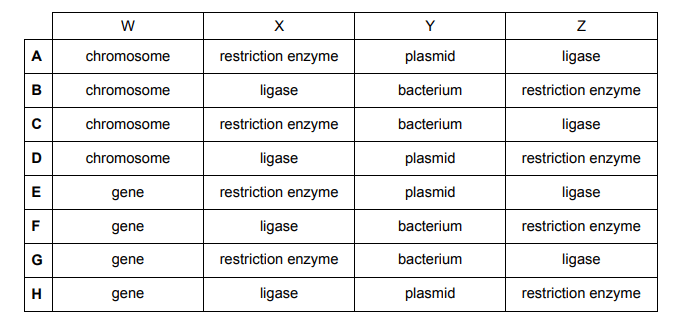1. Which row correctly identifies W, X, Y and Z?
3
0

## Explanation

This is a typical genetic engineering question which can be answered mostly through elimination.

W (the grey rectangle) is a small section of the chromosome shown in the picture – this is known as a gene. Hence, we can eliminate options A-D.

X appears two times in the picture and both times it is involved with cutting. This should indicate to you that X is a restriction enzyme. It is first used to cut the gene out of the chromosome and then the same enzyme used to cut the same section out of the plasmid. We are now down to options E and G which are only differentiated by Y.

Y is a plasmid as opposed to a bacterium. A plasmid is a circular strand of DNA present within the bacterium. It is the plasmid that gets cut by the restriction enzyme NOT the bacterium.

Z is seen to be sticking the gene taken out of the chromosome and the plasmid together. This indicates that Z is a DNA ligase which is the gluing enzyme.

Post Comment

A cleaning solution is used to remove limescale, CaCO3, from bathroom surfaces. When the solution is sprayed onto the limescale, effervescence (fizzing) occurs and the solid limescale begins to disappear. This chemical reaction is needed because the limescale cannot be removed with water alone.

1 The cleaning solution is acidic.

2 The effervescence (fizzing) is caused by the release of hydrogen gas.

3 The pH of the reacting solution will go down as the reaction proceeds.

4 The salt produced in the reaction is more soluble than CaCO3.

4
1

## Explanation

The first thing that you should notice is that CaCO3 is a metal carbonate. This should make you think of the general equation: metal carbonate + acid -> metal salt + water + carbon dioxide. The question states that when the cleaning solution is applied, there is effervescence (fizzing) which coincides with the release of carbon dioxide gas seen in the equation.

Hence, we can be confident that the cleaning solution is acidic which means that Option 1 is true.

The gas release, as mentioned earlier, is carbon dioxide gas NOT hydrogen so Option 2 is incorrect.

This is a neutralisation reaction as CaCO3 is a base (alkaline) and the cleaning solution is acidic. This means that as the reaction progresses, the pH gets closer to neutral i.e. the pH decreases as originally because of the presence of the base, the pH was high.  Therefore, Option 3 is incorrect.

Finally, although we don’t know what salt is produced at the end of the reaction, it says in the question that the solid limescale disappears.

This suggests that it is being converted into a more soluble salt. Hence, Option 4 is correct.

Post Comment

The diagram shows the forces acting on a cyclist as she accelerates at 4.0 m s–² on a straight, horizontal section of road. The constant resistive forces are air resistance and a 300 N force due to friction.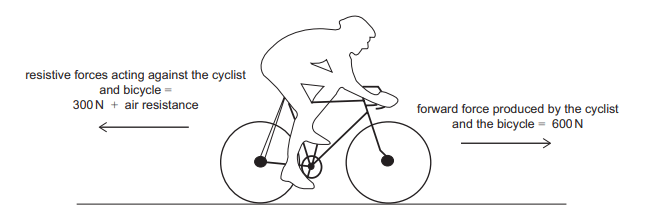The combined mass of the cyclist and bicycle is 50 kg.

3. What is the value of the air resistance?
2
0

## Explanation

We are given the values for mass and acceleration in the question which enables us to use the formula F=ma to calculate the resultant force of the cyclist. Remember that resultant force is the overall/net force of the cyclist

The resultant force is therefore 50 x 4 = 200N

Taking the direction the cyclist is travelling in as positive, we can then set up an equation where:  Forward force produced by the cyclist and the bicycle (600N) – Resistive forces acting against the cyclist and bicycle (300N) – Air resistance = 200N

600 – 300 – Air resistance = 200

Air resistance therefore = 100N, so B is the correct answer

Post Comment

The non-zero numbers p and q are such that p + q = 3( p – q)

What is the value of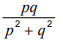4.
1
0

## Explanation

The first thing to do is simplify the equation given in the question p + q = 3(p – q) can be written as p + q = 3p – 3q

This can then be rearranged to 4q = 2p

Both sides can then be divided by 2 to simplify further to get p = 2q

We can now substitute the expression p = 2q into the equation the question is asking us to solve. Everytime p appears in this equation, we can simply replace this with 2q.

Numerator: 2q x q =2q²

Denominator (2q)² + q² = 4q² + q² = 5q²

Putting it all together: 2q²/5q² can then be simplified to ⅖

Post Comment

The labels 1, 2 and 3 in the following diagram represent cellular processes.

The shaded areas represent processes that can happen without the use of energy provided by cellular respiration.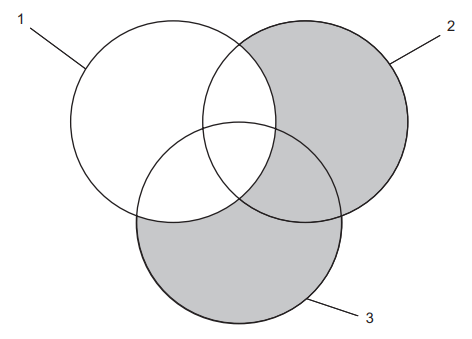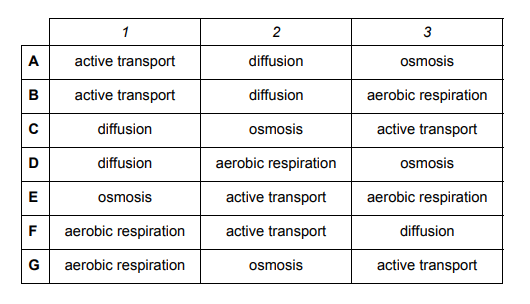5. Which processes could labels 1, 2 and 3 represent?
1
0

## Explanation

The question states that ‘the shaded areas represent processes that can happen without the use of energy provided by cellular respiration.’

This means that the processes within the shaded areas do not require ATP and are therefore passive processes.

Looking at the answer options, the processes mentioned in the table are active transport, diffusion, osmosis and aerobic respiration. We can sort these processes out into whether they require ATP or not as this will guide us towards the answer

 Requires ATP Does NOT require ATP Active transport Diffusion Aerobic respiration Osmosis

Area 1 is unshaded which suggests that the processes in this area are active i.e. require ATP. This means that active transport and aerobic respiration are the only processes that can be in Area 1. From this we can eliminate options C, D and E.

Areas 2 and 3 are shaded so the processes in these areas do not require ATP. This means that Areas 2 and 3 can contain diffusion and osmosis. From this we can eliminate options B, F and G. Therefore, A is the correct answer.

Post Comment

A chemist used electrolysis to electroplate a pure copper rod with a layer of silver. The concentration of metal ions in the electrolyte was kept constant.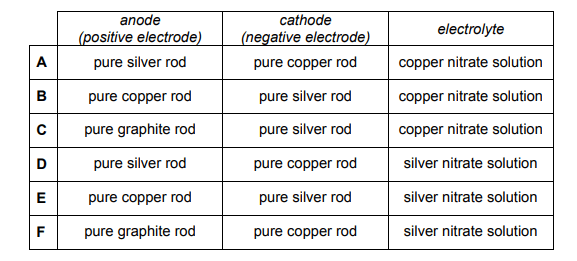6. Which row in the table identifies appropriate substances used as the anode (positive electrode), the cathode (negative electrode) and the electrolyte?
1
0

## Explanation

During electrolysis, positive ions move towards the cathode (the negative electrode) and get reduced i.e. they GAIN electrons. The question states that the chemist wants to electroplate a copper rod with a layer of silver – this means that silver is being deposited on the copper rod.

Therefore one of the half equations must result in the formation of silver atoms from their ions i.e. Ag+ + e- -> Ag

This is a reduction reaction so it will be happening at the cathode. This suggests that the cathode will be made of copper. Hence, we can eliminate options B, C and E.

The positive silver ions must therefore be coming from the anode which is therefore made of silver. The silver anode undergoes an oxidation reaction i.e. they LOSE electrons

Ag -> Ag+ + e-

We are now down to options A and D which are differentiated by the electrolyte. In electroplating, the electrolyte should be an aqueous solution of the coating metal. Therefore the electrolyte should be silver nitrate solution which makes option D the correct one.

Post Comment

A measuring cylinder resting on an electronic balance contains a liquid as shown in diagram 1.

A small solid object X is gently lowered into the liquid and no liquid splashes out of the cylinder.

The result is shown in diagram 2.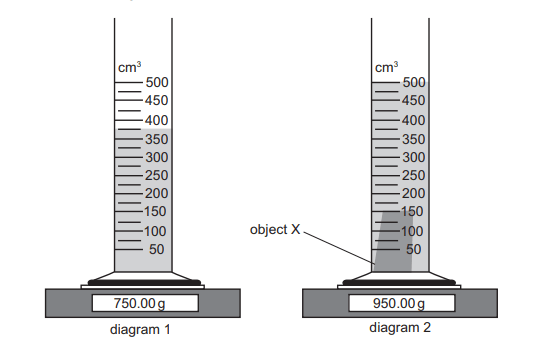7. What is the density of the material from which object X is made?
2
0

## Explanation

The formula for density is mass/volume

We can calculate the mass of the object X by finding the difference between the two values shown on the weighing scale. The mass of object X is therefore 950g – 750g = 200g

In a similar way, we can also find the volume of X. The value on the measuring cylinder when X has been lowered into the liquid is 500cm³.

The original level of the measuring cylinder was 375cm³. Therefore the volume of X is 500cm³ – 375cm³ = 125cm³

Inputting these values into the density formula, the density of X is 200/125 which is 1.6gcm

Post Comment

Find the value of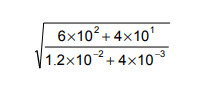8.
1
3

## ExplanationThe first thing to do is get rid of the standard form and write out the fraction as you normally would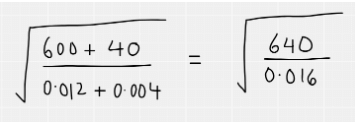After this, rewrite the fraction in terms of square numbers as these can then be easily square rooted. Remember that square rooting a fraction means multiplying each of the indices by a half. The fraction can then be simplified – note that when dividing indices, you simply subtract the indices from each other.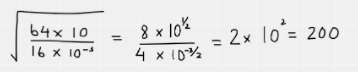Post Comment

A lizard was placed in a chamber with a constant flow of air. The temperature of the chamber was varied between 25°C and 10 °C. Apart from temperature and time, all other variables were kept constant.

The carbon dioxide concentrations in the air leaving the chamber are shown in the graph.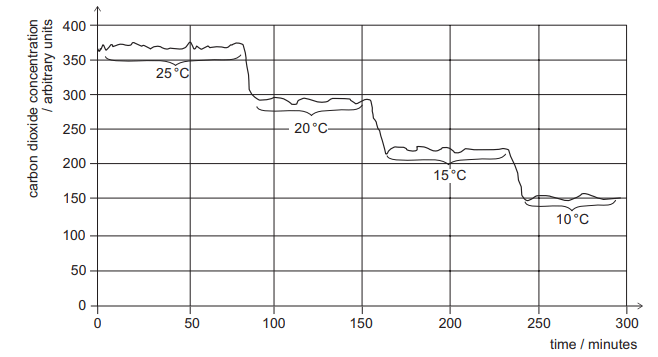1 Between 25 °C and 10 °C the respiration rate of the lizard is inversely proportional to the environmental temperature.

2 The data demonstrates that the lizard is only respiring aerobically.

3 The carbon dioxide could be detected by passing the air leaving the chamber through limewater.

9. Which of the following statements is/are correct?
2
0

## Explanation

Statement 1 suggests an inverse relationship between the respiration rate of the lizard and the environmental temperature i.e. that the respiration rate increases as the temperature drops from 25 degrees to 10 degrees. This is INCORRECT. We can see that the carbon dioxide concentration decreases as the temperature decreases – suggesting that the respiration rate of the lizard is decreasing. This is indicative of a directly proportional relationship NOT an inverse one.

Statement 2 is incorrect as we do not have enough information to conclude that the lizard is only respiring aerobically

Statement 3 is correct as the chemical test for the presence of carbon dioxide is by bubbling it through limewater.

Post Comment

Consider the non-radioactive elements in Group 1.

1 It has the most vigorous reaction with cold water.

2 Its atom has the same number of electrons in its highest occupied energy level as other members of the group.

3 It is the most readily oxidised.

10. Which of the following statements is/are correct about the element with the largest atomic number?
0
0

## Explanation

This question requires knowledge about the properties of Group 1 metals but can also be largely worked out through common sense. As you go down the group, the outer shell electrons are further away from the positively charged nucleus, experience more shielding and are subsequently less attracted to the nucleus and easier to lose. This means that the Group 1 metal with the largest atomic number (which is right at the bottom of the group) is the most reactive of them and thus the most easily oxidised (loses its outer electron the most readily). Thus, Statements 1 and 3 are correct. Statement 2 is also correct as all the Group 1 metals have one electron in their outer shell (outer shell is the same thing as the highest occupied energy level mentioned in the question).

Post Comment

A large metal sphere mounted on an insulating stand is negatively charged. A smaller, uncharged metal sphere mounted on an insulating stand is placed near to the negatively charged sphere.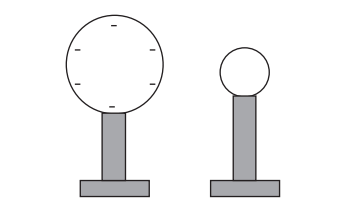The smaller sphere is connected to the Earth by a conducting wire. Electrons flow from the smaller sphere to Earth because they are repelled by the larger sphere. The smaller sphere is then disconnected from the Earth.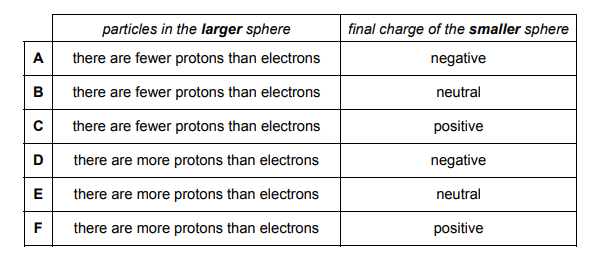11. Which row must be correct about the particles in the larger sphere and the final charge of the smaller sphere?
1
0

## Explanation

The question states that the larger sphere is negatively charged. This would suggest that there are fewer protons than electrons in the larger sphere to give an overall negative charge. Hence we can eliminate options D, E and F.

As electrons in the smaller sphere are repelled from the larger sphere and flow into the earth, the smaller sphere loses electrons. This will give it an overall positive charge. Therefore the correct option is C.

Post Comment

Point M has coordinates (6, 3p−1) and point N has coordinates (1−p, 2).

The gradient of the straight line joining M and N is −3 and it crosses the y-axis at (0, r).

12. What is the value of r ?
1
0

## Explanation

The general formula for working out a gradient is as follows. As we are told the co-ordinates for M and N, and the gradient, we can form an equation by substituting the coordinate values into the equation below.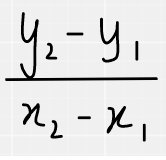Substitution of the coordinates looks like this: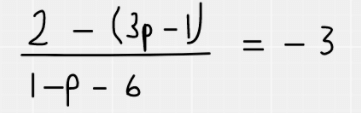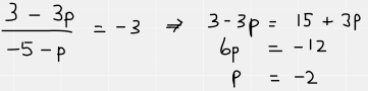We have now worked out that p = -2.

The point r is the y-intercept of the equation of the line

So using the format y = mx + c, the equation of the line is y = -3x + r

We can now input the actual co-ordinates for point M into y = -3x + r to find out the value of R

Having worked out that p = -2, the coordinates of M are (6, -7)

Therefore, -7 = -18 + r

r therefore = 11

Post Comment

1 amino acids in an animal

2 cellulose in a plant cell wall

3 lipids in a bacterial cell membrane

13. Which of the following is/are included in the carbon cycle?
1
0

## Explanation

Amino acids, Cellulose and Lipids all contain carbon and are ingested by other organisms. Hence they all make up part of the carbon cycle.

Post Comment

The decomposition of hydrogen peroxide in the presence of a manganese(IV) oxide catalyst produces water and oxygen gas.

2H2O2(aq) → 2H2O(l) + O2(g)

0.2 g of manganese(IV) oxide granules are added to 50 cm³ of 0.1 mol dm–³ hydrogen peroxide at 20 °C. The volume of gas collected is shown on the graph as curve X.

A second experiment is carried out at 20 °C using the same mass of manganese(IV) oxide.

The volume of gas collected is shown as curve Y.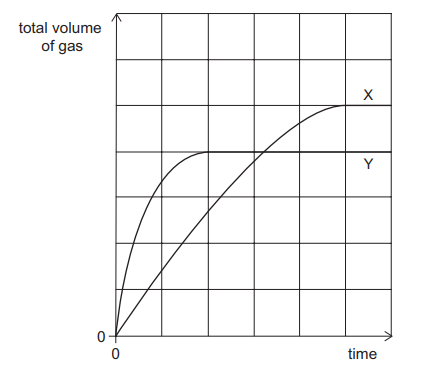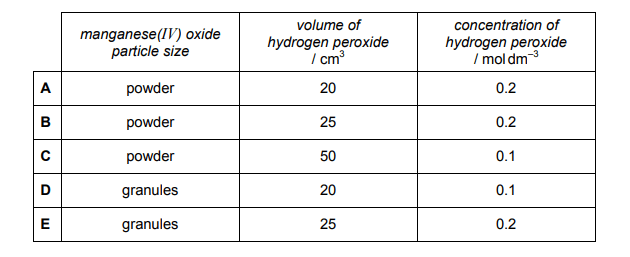14. Which of the following conditions could result in curve Y?
1
0

## Explanation

Comparing curve Y to curve X, the first thing that is noticeable is that Y has a steeper gradient to begin with, which implies a higher rate of reaction. This would suggest that the manganese(IV) oxide particle size is smaller in reaction Y i.e. it is a powder (as a high surface area gives rise to a higher rate of reaction). We can therefore eliminate options D and E.

The next thing to notice is that curve Y plateaus at a lower level than curve X. By counting the number of squares on the y axis we can work out how much gas is produced by reactions X and Y. Reaction X produced 5 squares worth of gas, whereas reaction Y only produces 4 i.e. Reaction Y produces ⅘ the amount of gas.

This would suggest that reaction Y uses ⅘ the amount of hydrogen peroxide compared to reaction X. Using the formula number of moles = concentration x volume, the number of moles of hydrogen peroxide used in Reaction X was 0.1 x 50/1000 = 0.005 mol. ⅘ of this amount is 0.004mol which is the number of moles of hydrogen peroxide used in Reaction Y.

We can then look at the remaining answer options A, B and C to see which two values multiply together to give 0.004. This shows that A is the correct answer.

Post Comment

Which statement about waves is correct?

15.
2
0

## Explanation

A is incorrect as longitudinal waves can travel at different speeds.

B is incorrect as it is transverse waves NOT longitudinal that cause vibrations perpendicular to the direction of energy transfer.

C is incorrect as sound waves are longitudinal

D is incorrect as in certain conditions, longitudinal waves can travel faster than transverse waves.

E is incorrect as all electromagnetic waves are transverse waves but this does not mean that all transverse waves are electromagnetic

F is correct as ultrasonic waves are a type of sound wave which are longitudinal

G is incorrect as ultraviolet waves are transverse waves so do not consist of compressions and rarefactions. Only longitudinal waves consist of these.

H is incorrect as X-rays can travel in a vacuum. All waves in the electromagnetic spectrum (transverse) can travel in a vacuum.

Post Comment

One side of a rectangle is (7− √5) cm.

The rectangle has an area of 66 cm²√.

16. What is the perimeter, in cm, of the rectangle?
2
0

## Explanation

We can draw the rectangle out as below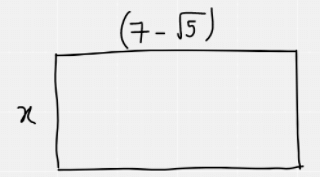The area of the rectangle is 66cm². So we can form the equation x(7-√5) = 66 and then rearrange it so that x is made the subject. As we have a surd in the denominator, we must rationalise which is why the numerator and denominator have both been multiplied by 7+√5. After this, there is some simplification of the fraction as 66 and 44 are both divisible by 22. We finally end up with a simplified expression for x.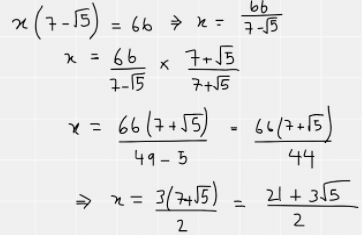The next thing is to work out the perimeter of the triangle.

This can be written as 2x + 2(7-√5)

As we now know the value for x, this is easy to do: 2x = 21 + 3√5

Therefore, perimeter = 21 + 3√5 + 14 – 2√5 which can then be simplified to 35 + √5, hence D is the correct answer.

Note that this is quite a time-consuming question so if you do notice you’re spending upwards of a minute and a half on this question and not nearing the answer, you may want to consider guessing and moving on.

Post Comment

A pea plant is heterozygous for seed colour and homozygous for seed shape.

Yellow seed colour (Y) is dominant to green seed colour (y). Round seed shape (R) is dominant to wrinkled seed shape (r).

1 This plant contains the alleles Y, y, R and r.

2 This plant contains only the alleles Y, y and R.

3 This plant contains only the alleles Y, R and r.

4 The genotype of this plant for seed colour is Yy.

5 The genotype of this plant for seed colour is YY.

6 The genotype of this plant for seed shape is Rr.

7 The genotype of this plant for seed shape is rr.

17. Using the information provided, which of the following statements, taken independently, could be correct?
0
0

## Explanation

The question states that the pea plant is heterozygous for seed colour which means that it has the genotype Yy. It also states the plant is homozygous for seed shape which means that it can either have the genotype RR or rr.

Statement 1 is incorrect as the plant cannot have both R and r alleles. It can only have one or the other.

Statement 2 could be correct.

Statement 3 is incorrect for the same reason as Statement 1.

Statement 4 is correct.

Statement 5 is incorrect as it is showing a homozygous genotype when the question states that seed colour is heterozygous.

Statement 6 is incorrect as it is showing a heterozygous genotype when the question states that seed shape is homozygous.

Statement 7 could be correct.

Post Comment

The concentration of Fe²+ ions in a solution can be determined by titration with acidifed potassium manganate(VII) solution.

The ionic equation for the reaction is:

5Fe²+ + MnO4–+ 8H+→ 5Fe³+ + Mn²+ + 4H2O

A 25.0 cm³ sample of solution containing Fe²+ ions reacted exactly with 10.0 cm³ of 0.0500 mol dm–³ potassium manganate(VII) solution.

18. What is the concentration of Fe²+, in mol dm–³ ?
0
0

## Explanation

The ionic equation shows that one mole of MnO4– ions reacts with 5 moles of Fe²+ ions.

Using the formula number of moles = concentration x volume, we can work out the number of moles of MnO4– ions that reacted with the iron ions.

Remember that volume in the formula is measured in dm³ so we must remember to convert

Moles of MnO4– ions = 10/1000 x 0.05 = 0.01 x 0.05 = 0.0005 mol = 5 x 10-4 mol

Therefore, the moles of Fe²+ ions is five times this amount = 2.5 x 10 mol

To work out the concentration = number of moles/volume. Convert both values to standard form to make the calculation easier.

Concentration of Fe²+ ions = 2.5 x 10/2.5 x 10 = 1 x 10-1 mol dm

Post Comment

Cosmologists have deduced that soon after the Universe was formed it was filled with electromagnetic radiation in the form of intense gamma-rays. They have also deduced that the Universe has been expanding since that time. One effect of this expansion is that the electromagnetic radiation that fills the Universe is now in the microwave region of the spectrum.

Here are three statements about the effects of the Universe expanding on the electromagnetic radiation that filled the early Universe:

1 The expansion has caused an increase in the frequency of the radiation.

2 The expansion has caused a decrease in the wavelength of the radiation.

3 The expansion has caused frequency to change in direct proportion to wavelength.

19. Which of the statements is/are correct?
0
0

## Explanation

The question states that the expansion has caused a change in EM radiation from gamma rays to microwaves. From your knowledge of the properties of EM waves, you should know that microwaves have a longer wavelength and lower frequency than gamma rays. Hence statements 1 and 2 are incorrect. Statement 3 is also incorrect as wavelength and frequency are always inversely proportional to each other.

Post Comment

To get to work, Sylvie first catches a bus and then catches a train.

The probability that the bus is on time is 0.6

The probability that the bus is late is 0.4

If the bus is on time, then the probability that she will catch the train is 0.8

If the bus is late, then the probability that she will catch the train is 0.6

20. Given that Sylvie catches the train, what is the probability that the bus was on time?
0
0

## Explanation

With probability questions like these, it is always useful to draw out a tree diagram.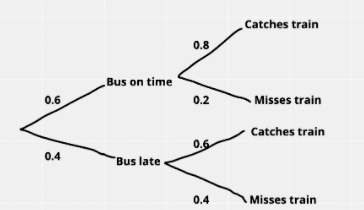The question contains the words ‘given that’ and so you should recall the ‘given that’ formula: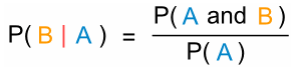The formula shows that the probability of event B happening given event A is equivalent to the probability of event A and event B divided by the probability of event A.

In this case, event A is Sylvia catching the train which is ‘given’ and event B is the bus being on time.

P(Bus on time | Catches the train) = (0.6 x 0.8)/((0.6×0.8) + (0.4 x 0.6))

= 0.48/0.48 + 0.24

= 0.48/0.72

= ⅔

Post Comment

1 A protein section coded for by part of a gene consisting of 500 base pairs will have a maximum of 166 amino acids.

2 A liver cell and a mature red blood cell from the same person will both have a chromosome number of 46.

3 The number of adenine bases in an allele must be the same as the number of guanine bases.

21. Which of the following statements is/are correct for a healthy human?
0
0

## Explanation

Statement 1 is correct as 3 base pairs code for 1 amino acid. Therefore 500 base pairs code for 166 amino acids as a maximum.

Statement 2 is incorrect as mature red blood cells do not have a nucleus and therefore do not have any chromosomes.

Statement 3 is incorrect as adenine is complementary to thymine so we would expect the same number of bases of adenine as thymine. This does not necessarily mean that guanine will have the same number of bases.

Post Comment

Complete combustion of 35 cm³ of a straight-chain alkane vapour gave 105 cm³ of carbon dioxide gas. Both gas volumes were measured at the same temperature and pressure.

22. Which of the following is the molecular formula of the alkane?
1
0

## Explanation

The question states that the vapour is an alkane which has the general formula CnH2n+2. As a result we are able to eliminate options A and C. Due to Avogadro’s Law which states that: 1 mole of every gas occupies the same volume, we can see that the carbon dioxide is being produced in a 3:1 ratio to the alkane vapour (as 105 = 35 x 3). Thus, for every molecule of alkane that is combusted, 3 molecules of carbon dioxide gas are produced. Therefore in terms of the molecular formula of the alkane, the number of carbons must be a multiple of 3 and hence option D is correct.

Post Comment

A water jet pack has lifted a person vertically upwards, as shown in the sketch. He is now stationary at a constant height.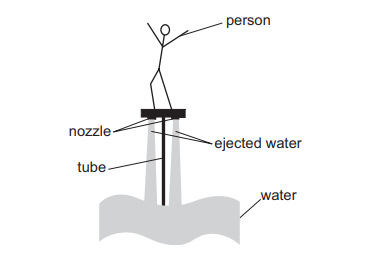Water rises through the tube and is ejected at a speed of 15 ms–¹ through two nozzles.

In a time of 12 s, each of the nozzles ejects 400kg of water vertically downwards.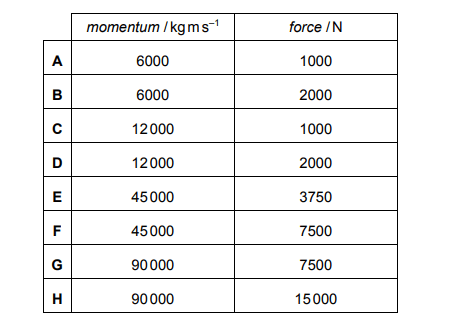23. What is the momentum of the water ejected by each nozzle in 12 s, and what is the upward force due to the water ejected by the jet pack?
1
1

## Explanation

The formula for momentum = mass x velocity

The mass is 400kg and the velocity is 15ms–¹

400 x 15 = 6000kg ms–¹

The formula which connects momentum and force is force = change in momentum/time

The initial momentum of the water we take to be 0 so the change in momentum is equivalent to 6000kg ms–¹.

Using the formula, the force is therefore 6000/12 = 500N

Since there are two nozzles on one jetpack, the total force exerted is 500 x 2 = 1000N

Post CommentAnon Medicmind Tutor

Tue, 26 Oct 2021 19:27:32

The answer says the momentum is 12000, but the answer in A is 6000N?- Medicmind Tutor

Sun, 31 Oct 2021 18:43:14

The question asks for the momentum of water exerted by *each* nozzle, so since each nozzle only ejects 400kg of water, the momentum for each nozzle would be m x v = 400 x 15 = 6000kgms^(-1). I think the explanation by Medicmind gives the change in momentum for both nozzles, which is only useful for finding the upward force in the second part of the question :)

A cross-country running track is in the shape of a regular pentagon.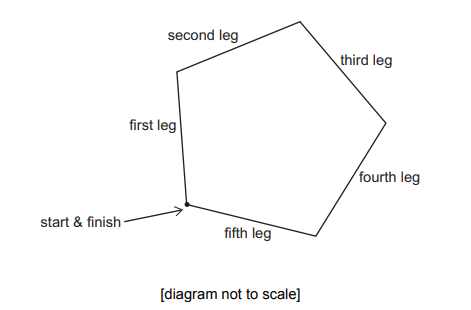Competitors run clockwise around the track.

When on the third leg of the course they run on a bearing of 110°.

24. What bearing do they run on for the first leg?
3
0

## Explanation

First, you must recall that the interior angle of a pentagon is 108 degrees.

The diagram below shows the bearing of 110 degrees at the start of the third leg. Focussing on this point and taking into account the interior angle, we can work out the angle marked in red is 142 degrees (360 – 110 – 108 = 142)

Due to the supplementary angles rule, we can work out the bearing of the second leg which is simply 180 -142 = 38 degrees.

Using the supplementary angles rule again, we can get the angle of 34 degrees (180 – (38 + 108) = 34)

Then to work out the bearing of the first leg it is 360 – 34 = 326 degrees.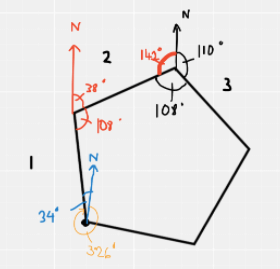Post Comment

A student carried out tests to find out which molecules were present in a sample of urine from a human male. The student recorded the presence of large protein molecules in the urine, and suggested that this indicated that the kidney was not functioning correctly.

1 damage to cell membranes between the blood vessels and the Bowman’s capsule

2 a reduced rate of active transport and selective re-absorption from the nephron

3 Cells in the collecting duct do not have receptors to bind to antidiuretic hormone (ADH) and so are unable to respond to the hormone.

25. Which of the following could have resulted in a high concentration of protein in the urine sample?
0
0

## Explanation

In the normal ultrafiltration process, large protein molecules should be unable to pass from the blood vessels into the Bowman’s capsule. There should be no protein in the ultrafiltrate. If there is protein in the urine, this is suggestive of a problem with the ultrafiltration process as nothing else could cause this. Hence Statement 1 is correct because if there is damage to cell membranes between the blood vessels and the Bowman’s capsule, this may mean that large proteins could pass through these damaged membranes into the capsule.

Statement 2 and 3 are both incorrect as they have no relevance to the ultrafiltration process.

Post Comment

Diborane has the formula B2H6.

Assume that boron consists of two isotopes, containing 20%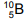atoms and 80%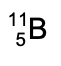atoms, and that all hydrogen atoms are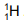.

Molecules of diborane will therefore have relative masses of 26, 27 or 28.

26. In what relative proportion will molecules of diborane with masses of 26, 27 and 28 occur?
0
1

## Explanation

Diborane molecules with a RMM of 26 are made up of two ¹ºB atoms (as well as 6 hydrogen atoms). The probability of this molecule forming based off the abundance of the isotopes given in the question is 0.2 x 0.2 = 0.04

Diborane molecules with a RMM of 27 are made up of one ¹ºB atom and one ¹¹B atom. Note that there are TWO combinations of these because you can get ¹ºB¹¹BH6 AND  ¹¹B¹ºBH6 . So the subsequent probability must be multiplied by two. The probability of this molecule forming is 2 x 0.2 x 0.8 = 0.32

Diborane molecules with a RMM of 28 are made up of two ¹¹B atoms. The probability of this molecule forming is 0.8 x 0.8 = 0.64

We can then create a ratio using these three probabilities

0.04 : 0.32 : 0.64

This can be simplified to 1: 8: 16

Post Comment

A 100% efficient ideal transformer has 400 turns on its primary coil and 100 turns on its secondary coil. The input voltage across the primary coil is 240V. An output current of 2.0A flows in the secondary coil.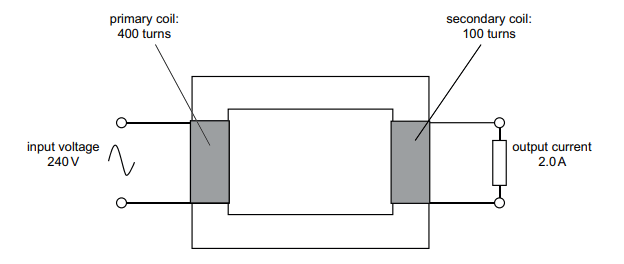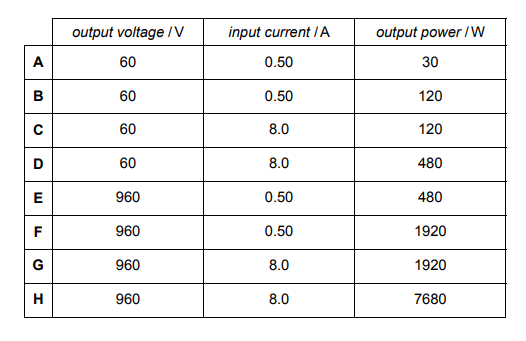27. What are the output voltage across the secondary coil, the input current in the primary coil, and the output power of the transformer?
0
0

## Explanation

This question incorporates all the formulae related to transformers.

The first formula to use involves the number of turns on the coils and voltage: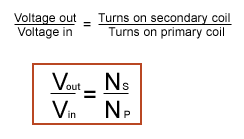We will represent voltage out as x

x/240 = 100/400

x = 60 V

Thus, we can eliminate options E, F, G and H

As the transformer is 100% efficient we can use the equation VpIp = VsIs to work out the input current. We will represent input current as y.

240 x y = 60 x 2

y = 0.5 A

We can further eliminate options C and D

Finally to work out output power, we use P = IV using the values for output voltage and current

Power = 2 x 60 = 120W

Therefore, the correct answer is B.

Post Comment

## Instructions

Below is a summary of your answers. You can review your questions in three (3) different ways.

The buttons in the lower right-hand corner correspond to these choices:

2. Review questions that are incomplete.
3. Review questions that are flagged for review. (Click the 'flag' icon to change the flag for review status.)

You may also click on a question number to link directly to its location in the exam.

## Instructions

This review section allows you to view the answers you made and see whether they were correct or not. Each question accessed from this screen has an 'Explain Answer' button in the top left hand side. By clicking on this you will obtain an explanation as to the correct answer.

At the bottom of this screen you can choose to 'Review All' answers, 'Review Incorrect' answers or 'Review Flagged' answers. Alternatively you can go to specific questions by opening up any of the sub-tests below.

x

## When should we call you?

It would be great to have a 15m chat to discuss a personalised plan and answer any questions

## What time works best for you? (UK Time)

Pick a time-slot that works best for you ?

Submit

Submit